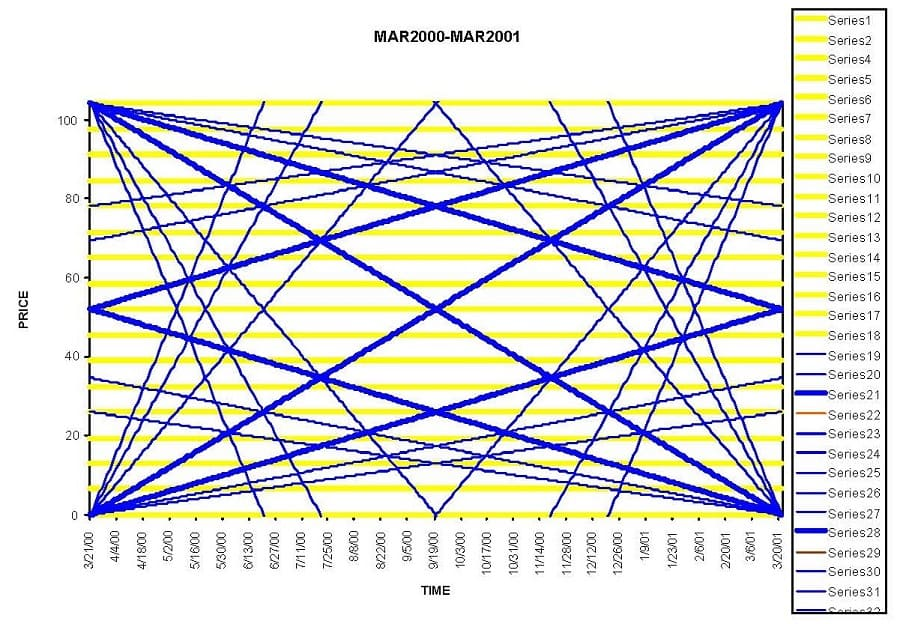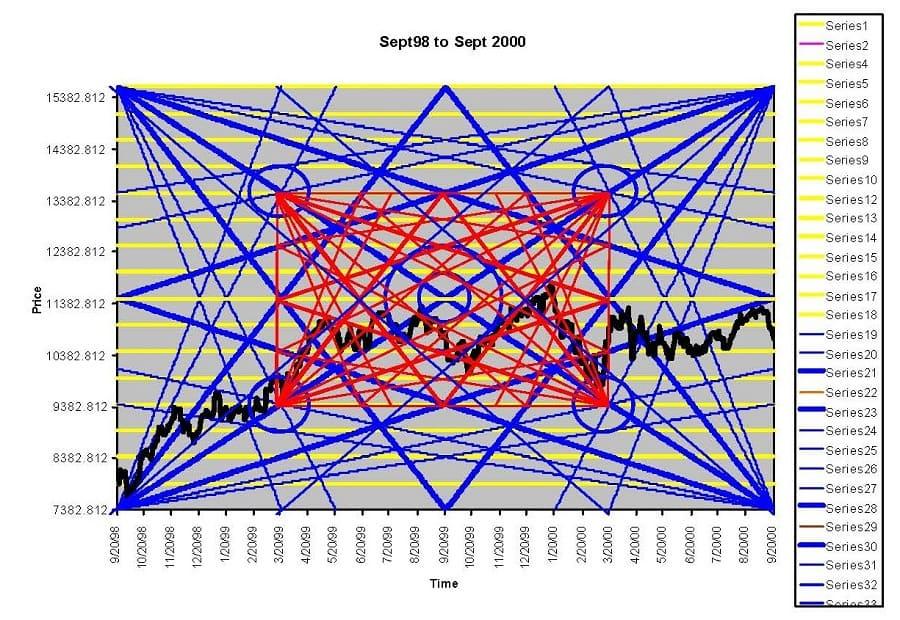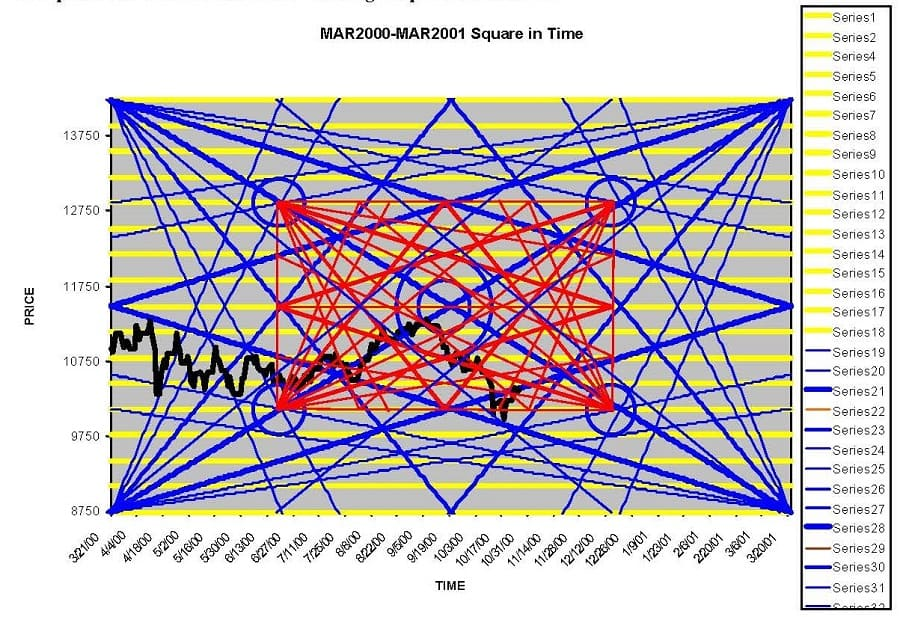# Gann’s Master Mathematical Price & Time & Trend Calculator

In 1954, at the age of nearly 76, W.D. Gann released a course based upon mathematical rules for making market predictions. In the advertisement for this method the author of the add writes: “We will not sell the course of instructions or teach the mathematical formula for market predictions just to get money, which we do not need or have to have; we will only sell the courses to people wh meet our requirements, and if we feel after a thorough investigation that a man or woman cannot make a success investing or trading we will refuse to teach them the courses”. “The Master Calculator can only be used with the Master Courses. It is not sold separate from the other courses”.

The price shown for Gann’s Master Course in this ad is \$5,000.00, it does not list the additional cost of the Master Mathematical Calculator. The ad describes the calculator as a plastic overlay that accurately measures Time, Price and Trend. Many Gann experts and students have tried to use these overlays, which require the chart to be hand drawn to a proper scale on graph paper that has 8 squares to one inch. This creates a lot of extra tedious work.

This has caused one of Gann’s greatest discoveries to have been overlooked for many years. I know that some software programs have the ability to make these overlays on price charts but that is still not going to help most people because it turns out that there are also very precise mathematical rules regarding the horizontal axis or Time! In fact, these same mathematical rules apply to price as well but we will leave this topic for latter. The advertisement itself contains clues about the calculator, such as “W.D. Gann’s Record for 52 years”, “After fifty-two years of experience and research going back hundreds of years, I have proved to my entire satisfaction that history repeats and that when we know the past, we can determine the future of prices”. The calculator that Gann is talking about in this ad is his square of 52 overlay, which is based upon the 52 weeks in a year. The basic overlay looks like this:This is also the same geometric form that Gann used for the Square of 90 and Square of 144 plastic overlays. This basic structure or overlay appears in many books on Sacred Geometry and is also the secret to George Bayer’s “Trend Determination” course. Getting back to Gann and the square of 52 course we find that this is the course where Gann made the confusing statement: “ Within the circle forms the square, there is an inner circle and an inner square, as well as an outer square and an outer circle which prove the Fourth Dimension in working out price movements”. This quote is describing a fractal nature or structural pattern existing in the markets that is the same on smaller levels as it is at larger levels.

\$17.50

\$46.30

\$22.30

### W. D. Gann Master Commodities Trading Course

\$25.00

Jumping over to Gann’s Angle course, on the last page, GA-32, Gann says: “Figuring \$100, or par, as a basis for stock prices and changing these prices to degrees, 12½ = 45-degrees, 25 = 90-degrees, 37½= 135-degrees, 50 = 180-degrees, 62½ = 225-degrees, 75 = 270-degrees, 82½ = 315-degrees, and 100 = 360”. This tiny little paragraph is the basis of the entire Murrey Math Trading Course, which readers of Traders World should be very familiar with since, T.H. Murrey has written numerous articles for the magazine.

These numbers are all natural 8th numbers off of the base of 10, 100, 1000, 10000, 100000, etc. The part that is most confusing is balancing this with the time element, but based on the information given by Gann himself it should be obvious that he has already shown us part of the relationship. For example: If shares of XYZ stock moved up 12½ dollars in 45 days, weeks or 45 months then the stock is on the price degree of it’s time angle, that is to say that price and time are equal or balanced. Gann’s example: “When a stock sells at 50 on the 180th day, week or month, it is on the degree of its time angle”.

“On February 1, 1915, U.S. Steel made a low at \$38, which is closest to a price of \$37½, which is 3/8th of 100 and equals 135-degree angle. Steel was 14 years or 168 months old on February 25, 1915, and hit the angle of 135-degrees, which showed that the price of Steel was behind time, but was in a strong position, holding at \$38 above the 135-degree angle or the price \$37½”. Just to make sure that you understand this information, U.S. Steel would have been balanced or “on the degree of its time angle” at a price of \$46 5/8th because \$100/ 360-degrees = 0.27777cents per degree and 168 months multiplied by 0.27777 = \$46.67 which is closest to \$46 or 5/8th in price. Therefore, Steel is \$8 5/8th behind time.

T.H. Murrey has his time rules based off the “Harvest Moon” but I would stick to W.D. Gann’s time definitions when using this natural 8th system and his plastic overlays as his method has a clear degree relationship to both price and time and also to the fractal structure, which he described in the quote mentioned above. I will discuss how to set up these charts properly in terms of time latter in the article with specific examples. Gann goes on to say:

“When Steel reached \$200, it equaled 2 circles of 360-degrees (two complete cycles of \$100). When it advanced to \$261¾, it was closest to \$62½ (5/8th) in the third cycle of \$100 or nearest the 225-degree angle or 5/8th point, which is the strongest angle after it crossed the half-way point at \$250 or the 180-degree angle”. Note that Gann rejects the two circles or cycles of \$100 price to convert the time angle. These instructions are also very different than what Murrey Math instructs students to do but I do not want to get into that subject.

Moving on to the subject of time we know that Gann placed great emphasis on the cardinal points of the solar year. These are the Vernal Equinox, Summer Solstice, Autumnal Equinox and Winter Solstice or March 21st, June 21st, September 21st, and December 21st respectively. Gann always said that the year begins at March 21st (not January 1st) and that this was a very important seasonal time. Now this is where Gann’s “within the circle forms the square” quote gets real interesting! If we treat each natural year (March 21st to March 21st) as a complete circle, which it is because the earth has gone 360-degrees around the Sun, and take W.D. Gann at his word that there is a square within this orbit then we come up with some very unique geometrical information about time and squares, i.e. Gann’s plastic overlays.

Lets build a hypothetical square running from Vernal Equinox to Vernal Equinox as our horizontal axis and we will use 0 to 100 as our vertical. If we draw in the two 45-degree Angles corner to corner we have a shape that looks like a box with an x in it. The two 45’s will meet at the exact center of both price and time at \$50 on the Autumnal Equinox or September 21st. If we bisect this square again with a horizontal line going left to right at \$50, and a vertical line going straight up from the Autumn Equinox we can create four smaller squares with half of the x already completed. If we complete these smaller boxes with the missing 45-degree line we will find that these points come out at \$25 and \$75 in price and also 25% and 75% in time or Sumer Solstice to Winter Solstice (June 21st to December 21st). If we take these 4 points, which are \$25 on June 21st, \$75 on June 21st, \$25 on December 21st and \$75 on Decembers 21st and make a new square, we find that our new square is exactly half the size of our former square set within the ¼ points in terms of both price and time.

Our new square also maintains the exact center of our old square at \$50. This new square is now an exact musical octave of the old square because it’s based upon powers of 2 or is exactly ½ our old square. This tells us that if we want to find a square that is within our old square that we will only find it between the two Solstice points in the year! If we wanted to enlarge the original square (to find an outer square) we would again follow the natural law of the musical octave, i.e. powers of 2. The first part of the problem is easy because we know that the next square is going to be twice as large as our old square. So this means that we are moving up from a one-year square to a two -year square in terms of time but this does not answer where in time this new square begins and ends. To calculate where this larger square begins and ends we have to use the rule “as above so below”.

In other words, when we made the smaller square, we found that it existed within the larger square at the 25% and 75% points in time, therefore March 21st to March 21st is within the ¼ points of the larger square above it. In our one-year square, a ¼ of time is 90-degrees or 91.3125 solar days. This means that a ¼ of time in the next larger square is 180-degrees or 182.625 solar days, i.e. twice as big. If we add and subtract 180-degrees/days from our smaller square running March to March, we will find that our two-year square begins and ends at the Autumnal Equinox. The same is true for a 4-year, 8-year, 16-year square, etc. Getting back to the inner squares, a 90-degree square (1/2 of our 180-degree Summer Solstice to Winter Solstice and winter back to summer circle) would begin and end 45-degrees after the Sumer Solstice and 45-degrees before the Winter Solstice because 45 is ¼ of 180. This would be 45.65 days or ¼ of 182.625. So this means we would have another (90-degree) square running inside the (180-degree) Solstice squares beginning on August 5th and ending November 5th.

Then just as we have two 180-degree Solstice squares running from Summer to Winter, then Winter back to Summer, we also have four 90-degree squares running from August 5th to November 5th , November 5th to February 5th , February 5th to May 5th and May 5th back to August 5th . These are all exact mathematical points to look for squares based upon Gann’s plastic overlay pattern. I am providing two charts of the Dow Jones Industrial Average (closing price only) so that you can visually see what Gann was describing in his Square of 52 course.“ Within the circle forms the square, there is an inner circle and an inner square, as well as an outer square and an outer circle which prove the Fourth Dimension in working out price movements”.Please note that the inner square is an exact musical octave of the larger outer square.

• Gann’s Master Mathematical Price & Time & Trend Calculator By Daniel T. Ferrera1.Kas says: